# Kendall coefficient of rank correlation

(diff) ← Older revision | Latest revision (diff) | Newer revision → (diff)

KendallOne of the empirical measures of dependence of two random variablesandbased on ranking the elements of the sample. Thus, the Kendall coefficient is a rank statistic and is defined by the formula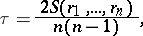whereis rank ofbelonging to the pair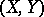for which the rank ofis equal to,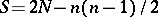,being the number of elements of the sample for which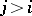and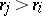simultaneously. The inequalityalways holds. The Kendall coefficient of rank correlation has been extensively used (see ) as an empirical measure of dependence.

The Kendall coefficient of rank correlation is applied for testing hypotheses of independence of random variables. If the hypothesis of independence is true, then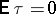and. For small samplesstatistical testing of hypotheses of independence is carried out by means of special tables (see ). When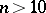the normal approximation for the distribution ofis used: If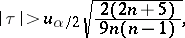then the hypothesis of independence is rejected and the alternative is accepted. Hereis the significance level, and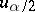is the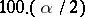-percent point of the normal distribution. The Kendall coefficient of rank correlation can be used for revealing dependence of two qualitative characteristics, provided that the elements of the sample can be ordered with respect to these characteristics. If,have a joint normal distribution with correlation coefficient, then its relation to the Kendall coefficient of rank correlation has the formHow to Cite This Entry:
Kendall coefficient of rank correlation. Encyclopedia of Mathematics. URL: http://encyclopediaofmath.org/index.php?title=Kendall_coefficient_of_rank_correlation&oldid=13189
This article was adapted from an original article by A.V. Prokhorov (originator), which appeared in Encyclopedia of Mathematics - ISBN 1402006098. See original article